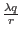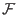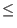Next: Mathematics Used in this Up: Quantum Computing, Shor's Algorithm, Previous: Glossary   Contents

# Glossary

This is a glossary of terms and variables used throughout this paper.: In the context of Shor's algorithm in integer such that m =.

a
: A variable that when used in the context of Shor's algorithm is an argument to the function(a) = xa mod n. It may be a single integer, or it may denote a superposition of states.

Classical computer
: A computer whose internal workings behave in manner consistent with classical physics. Data registers in a classical computer can not exist in a superposition of states. By definition (e.g. the Church-Turing Thesis) a classical computer can only compute functions that a Turing machine can compute.

Classical physics
: The model that was used to describe physical phenomenon before the advent of quantum physics. The predictions of classical physics with regard to the behavior of fundamental particles are incorrect.

Collapse
: A collapse in the context of this paper is what happens to the state vector of a quantum mechanical system when that system is observed or measured. Since the system can only be measured to be in one of its base states, the state vector will collapse from some superposition of base states into the measured state only.

Complex vector space
: A vector space in which the coordinates of a vector are complex numbers.

Complexity class
: A grouping of algorithms based on how their memory usage and number of operations scale with the size of the input.

Coprime
: Integers a and b are coprime if their greatest common denominator is one.

Discrete Fourier Transform
: A transformation converts a finite list of equally spaced samples of a function into a list of coefficients of finite combinations of circles, ordered by their frequencies, that have the same values. In Shor's algorithm it is used to calculate and multiple of the inverse period, where the period is the quantity which enables Shor's algorithm to find factors of a number n.

gcd
: This is an abbreviation for the mathematical function which calculates the greatest common denominator of two integers. The greatest common denominator of two integers a and b is the largest integer c such that a/c and b/c are integers.

Hilbert Space
: A complex linear vector space. The complete state of a n state quantum mechanical system can be represented by a vector in an n dimensional Hilbert Space.

Linear vector space
: A vector space such that vectors within the space which are added or multiplied together result in vectors that also lie within the same space.

Memory register
: A array of bits on a classical computer. A memory register of size n may store one of 2n values.

n
: In the context of Shor's algorithm, a number to be factored.

Periodic function
: A function with a period r such that(x) =(x + r) =(x + 2r) and so on. Sine and Cosine are typical examples of periodic functions.

q
: In the context of Shor's algorithm the power of 2 such that n2q < 2n2.

Quantum memory register
: A array of n qubits which can exist in any superposition of its 2n base states.

Quantum parallelism
: The ability of a quantum computer to perform an operation on a quantum memory register which results in the simultaneous calculation of a function function of 2n different values where n is the size of the quantum memory register.

Quantum physics
: Currently the most complete model for describing the behavior of small physical systems.

Qubit
: A two state quantum mechanical system, which can exist in any superposition of the 0 and 1 state. In this paper I have considered a spin-1/2 particle as a possible candidate for a qubit in a physical implementation of a qubit.

r
: In the context of Shor's algorithm the period of the periodic function xa mod n.

Shor's Algorithm
: A algorithm designed by Peter Shor of Bell Labs which finds factors of a number n in polynomial time relative to the number of bits in n's binary representation on a quantum computer. The fastest published algorithm on a classical computer is slower than polynomial time, and the presumed intractability of this problem is the basis for many cryptographic systems.

Spin-1/2 particle
: A particle which can be characterized as having a spin of +1/2 or -1/2. Examples include the proton, neutron, and electron.

State vector
: In the context of this paper, the state vector in a Hilbert Space which completely describes a quantum mechanical state vector - such as the state of quantum memory register.

Superposition of states
: A mixture of base states. The state vector for a quantum mechanical systems which can be measured in one of n base states can exist as any combination of components of the base states.

x
: In the context of Shor's algorithm a integer which is coprime to n and used in the function(a) = xa mod n.Next: Mathematics Used in this Up: Quantum Computing, Shor's Algorithm, Previous: Glossary   Contents
Matthew Hayward - Quantum Computing, Shor's Algorithm, and Parallelism GitHub Repository